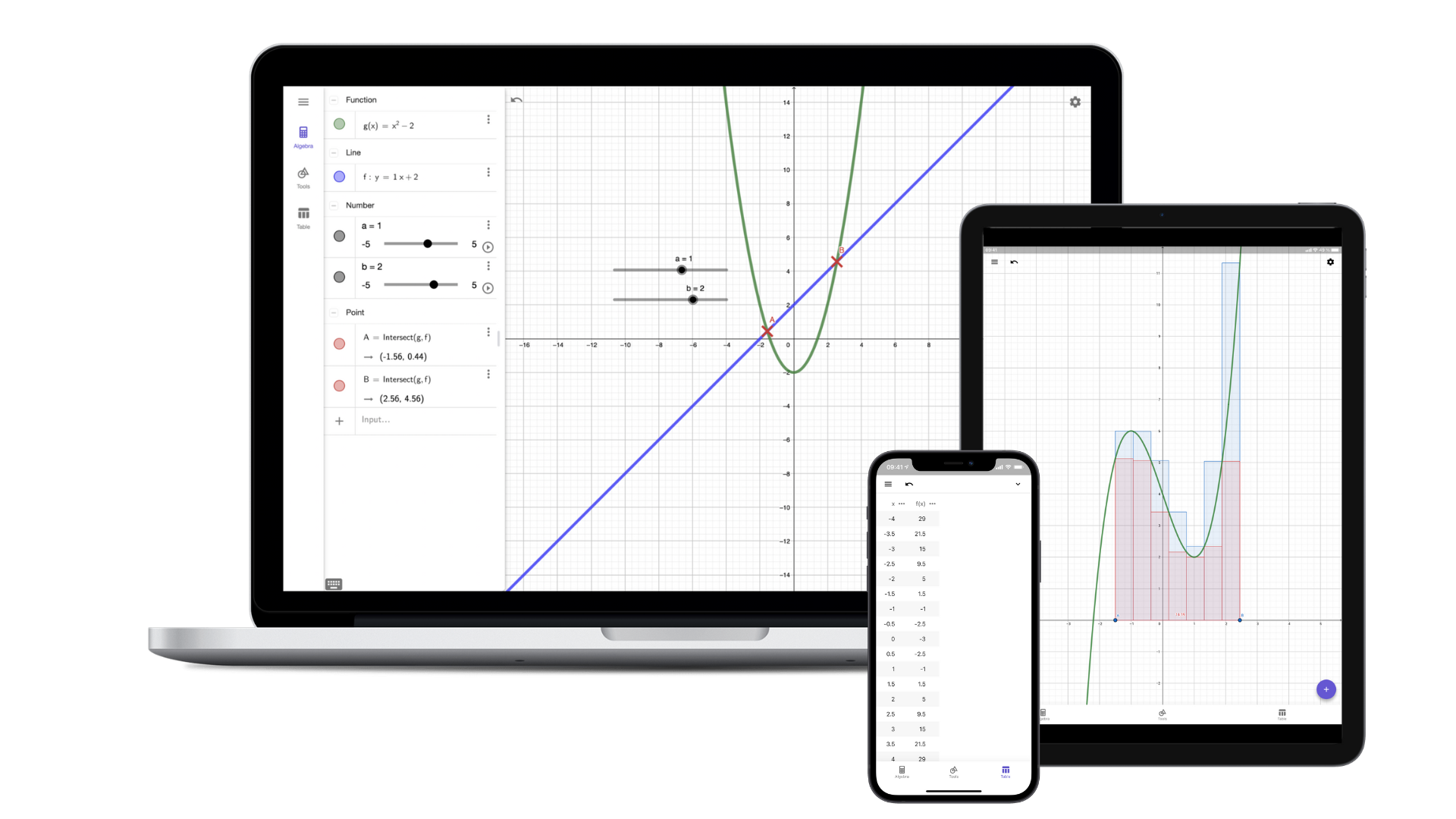# Graphing Calculator

## Introducing the Graphing Calculator

The GeoGebra Graphing Calculator is a powerful and interactive math app designed for visualizing and analyzing functions, equations, and inequalities in 2D.

It enables you to explore mathematical relationships, create graphs, find special points, and understand key concepts in algebra, calculus, and statistics. This user-friendly tool promotes a deeper understanding of math concepts and fosters an engaging learning experience for students and educators alike.The Graphing Calculator comprises the following components:

• Graphics View: Showcases the visual representation of all created objects, such as points and graphs, providing an interactive canvas for exploring mathematical relationships.
• Algebra View: Displays the algebraic representations of all created objects, like coordinates and equations, and enables you to enter algebraic expressions or commands.
• Tools View: Offers a range of essential tools for constructing points, lines, and vectors, as well as performing various actions like zooming and altering the appearance of objects.
• Table View: Generates a table of values for functions, allowing you to observe and analyze numerical relationships between variables.

These components work together to create a dynamic and engaging environment for learning and exploring math concepts using the Graphing Calculator. Discover the full potential of the GeoGebra Graphing Calculator by exploring our official tutorial, complete with step-by-step instructions to guide you through its features and functionalities.